Copyright (C) 2012-present, The Computational Data Science Lab
https://github.com/cdslaborg/paramonte
References

# Sampling a univariate normal distribution via the ParaMonte library's ParaDRAM routine

NOTE
If you are viewing an HTML version of this MATLAB live script on the web, you can download the corresponding MATLAB live script *.mlx file to this HTML page at,
This code, along with other MATLAB live scripts, is located at,
https://github.com/cdslaborg/paramontex/tree/main/MATLAB/mlx
Once you download the file, open it in MATLAB to view and interact with its contents, which is the same as what you see on this page.
NOTE
This ParaDRAM sampler example is also available in Python language as a Jupyter Notebook, on this page,
This Jupyter Notebook, along with other Python Jupyter Notebooks, is located at,
https://github.com/cdslaborg/paramontex/tree/main/Python/Jupyter
IMPORTANT - macOS users
If you are a macOS (Darwin) user and you have downloaded the prebuilt ParaMonte libraries from the GitHub release page to use on your macOS system, read this troubleshooting page before continuing.

## Setting up the path to the ParaMonte::MATLAB library

================================================================
First, we will clean up the MATLAB environment and make sure the path to the ParaMonte library is in MATLAB's path list.
clc;
clear all;
close all;
format compact; format long;
%%%%%%%%%%%% IMPORTANT %%%%%%%%%%%%%
% Set the path to the ParaMonte library:
% Change the following path to the ParaMonte library root directory,
% otherwise, make sure the path to the ParaMonte library is already added
% to MATLAB's path list.
pmlibRootDir = './';
%%%%%%%%%%%%%%%%%%%%%%%%%%%%%%%%%%%%
% change MATLAB's working directory to the folder containing this script
% if MATLAB Live Scripts did not create a temporary folder, we would not
% have all of these problems!
try
setwdFilePath = websave("setwd.m","https://github.com/cdslaborg/paramontex/raw/main/MATLAB/mlx/setwd.m");
run(setwdFilePath); % This is a MATLAB script that you can download from the same GitHub location given in the above.
catch % alas, we will have to run the simulations in MATLAB Live Script's temporary folder
filePath = mfilename('fullpath');
[currentDir,fileName,fileExt] = fileparts(filePath); cd(currentDir);
cd(fileparts(mfilename('fullpath'))); % Change working directory to source code directory.
end
Suppose we want to sample random points from a standard univariate Gaussian function. The following MATLAB function getLogFunc() returns the natural logarithm of the Probability Density Function of the univariate standard Gaussian distribution.
NDIM = 1; % number of dimensions of the domain of the objective function
logSqrt2Pi = log(sqrt(2*pi));
getLogFunc = @(x) -0.5 * x.^2 - logSqrt2Pi; % the objective function
NOTE
Since the mathematical objective functions (e.g., probability density functions) can take extremely small or large values, we often work with their natural logarithms instead. This is the reason behind the naming convention used in the ParaMonte library for the user's objective functions: getLogFunc, implying that the user must provide a function that returns the natural logarithm of the target objective function.
See MATLAB Live Script for an in-depth discussion of why we need to work with the logarithm of mathematical objective functions in optimization and sampling problems.
IMPORTANT
Note that in MATLAB all vectors and arrays are, by default, column-major, and so is the input value, point, to getLogFunc(). This means that the size of point is (ndim,1). Thus, all other vectors that interact with point, for example, the mean vector, must be also of the same size as point: (ndim,1).

## Running a ParaDRAM simulation on a single processor

==================================================================
We will sample random points from this objective function by calling the ParaDRAM sampler (Delayed-Rejection Adaptive Metropolis-Hastings Markov Chain Monte Carlo sampler) of the ParaMonte library. The simplest scenario would be to simulate with the default specifications that are appropriately determined by the ParaDRAM sampler. However, for further clarity of this particular example, we will specify an output folder for the automatically-named output files of the simulation and fix the random number generator's seed for reproducibility.
NOTE
To run the sampler in parallel, you will have to first save the MPI-enabled script as an external file. Visit the ParaMonte library's documentation website for more information.
We will first create an instance of the paramonte class and then, a ParaDRAM sampler instance from that,
pm = paramonte();
pm.verify();
::::::::::::::::::::::::::::::::::::::::::::::::::::::::::::::::::::::::::::::::::::::::::::::: :::: :::: _/_/_/_/ _/_/ _/_/ _/ _/ _/_/_/_/_/ _/ _/ _/ _/_/_/_/ _/ /_/_/ _/_/_/_/ _/ _/ _/ _/_/ _/_/_/ _/_/_/ _/_/_/ _/_/_/ _/ _/ _/_/ _/ _/ _/ _/ _/ _/ _/ _/ _/ _/_/_/_/ _/ _/ _/ _/ _/ _/ _/ _/ _/ _/ _/ _/ _/ _/ _/_/_/ _/_/_/_/ _/ _/_/_/_/ _/_/_/ _/_/_/ _/_/ _/ _/ _/_/ _/_/_/ ParaMonte plain powerful parallel Monte Carlo library Version 2.3.0 :::: :::: ::::::::::::::::::::::::::::::::::::::::::::::::::::::::::::::::::::::::::::::::::::::::::::::: ParaMonte - NOTE: Intel MPI library for 64-bit architecture detected at: ParaMonte - NOTE: ParaMonte - NOTE: "C:\Program Files (x86)\IntelSWTools\compilers_and_libraries_2020.1.216\windows\mpi\intel64\bin" ParaMonte - NOTE: ParaMonte - NOTE: To perform ParaMonte simulations in parallel on a single node, run the ParaMonte - NOTE: following two commands, in the form and order specified, on a MATLAB-aware ParaMonte - NOTE: mpiexec-aware command-line interface, such as the Intel Parallel Studio's command-prompt: ParaMonte - NOTE: ParaMonte - NOTE: "C:\Program Files (x86)\IntelSWTools\compilers_and_libraries_2020.1.216\windows\mpi\intel64\bin\mpivars.bat" ParaMonte - NOTE: ParaMonte - NOTE: mpiexec -localonly -n 3 matlab -batch "main_mpi" ParaMonte - NOTE: ParaMonte - NOTE: where, ParaMonte - NOTE: ParaMonte - NOTE: 0. the first command defines the essential environment variables and, ParaMonte - NOTE: the second command runs in the simulation in parallel, in which, ParaMonte - NOTE: 1. you should replace the default number 3 with the number of ParaMonte - NOTE: processors you wish to assign to your simulation task, ParaMonte - NOTE: 2. main_mpi.m is the MATLAB file which serves as the entry point to ParaMonte - NOTE: your simulation, where you call the ParaMonte sampler routines. ParaMonte - NOTE: ATTN: Notice the MATLAB filename must appear without the file extension. ParaMonte - NOTE: ATTN: Notice the MATLAB filename must be enclosed with quotation marks. ParaMonte - NOTE: 3. -localonly indicates a parallel simulation on only a single node (this ParaMonte - NOTE: flag will obviate the need for MPI library credentials registration). ParaMonte - NOTE: For more information, visit: ParaMonte - NOTE: ParaMonte - NOTE: https://software.intel.com/en-us/get-started-with-mpi-for-windows ParaMonte - NOTE: ParaMonte - NOTE: Note that the above two commands must be executed on a command-line that recognizes ParaMonte - NOTE: both MATLAB and mpiexec applications, such as the Intel Parallel Studio's command-prompt. ParaMonte - NOTE: For more information, in particular, on how to register to run Hydra services ParaMonte - NOTE: for multi-node simulations on Windows servers, visit: ParaMonte - NOTE: ParaMonte - NOTE: https://www.cdslab.org/paramonte ParaMonte - NOTE: To check for the ParaMonte or the MPI library installations status or, ParaMonte - NOTE: to display the above messages in the future, use the following ParaMonte - NOTE: commands on the MATLAB command-line: ParaMonte - NOTE: ParaMonte - NOTE: pm = paramonte(); ParaMonte - NOTE: pm.verify();
% the forward-slash below indicates that the provided string should be treated
% as the folder name and not as the prefix for the simulation output files.
pmpd.spec.outputFileName = "out/gaussian";
pmpd.spec.overwriteRequested = true; % overwrite the simulation output files if they already exist
% we will also request a much smaller number of uniquely-sampled points (5000)
% than the sampler's default value (100000).
pmpd.spec.chainSize = 5000;
% initialize the random seed to generate reproducible results
pmpd.spec.randomSeed = 31951;
pmpd.runSampler ( 1 ... This is the number of dimensions of the objective function
, getLogFunc ... This is the objective function
)

## General Guidelines on the ParaMonte visualization tools

===================================================================
In the following sections, we will discuss some of the postprocessing and visualization tools that automatically ship with the ParaMonte library. However, this is just the tip of the iceberg. For more detailed instructions on how to use the individual visualization tools of the ParaMonte library, visist the following MATLAB live scripts,
line / scatter plots
density plots
other plots
This will print the realtime simulation progress information on your MATLAB prompt window (if you are on a Windows system) and on the Bash terminal from which you invoked the matlab command to open MATLAB. Once the simulation is finished, the ParaDRAM routine generates a number of output files in the folder specified by the simulation specification variable pmpd.spec.outputFileName. Each of the output files contains information about certain aspects of the simulation. To understand the contents and utilities of these files, simply open them to look at their contents or visit this page:
Upon finishing the simulation, the sampler provides hints on how to postprocess the results. Now, we can read the generated output sample, contained in the file suffixed with *_sample.txt.
sample = sample{1};
To quickly visualize the generated sample as a histogram, try,
sample.plot.histogram.make();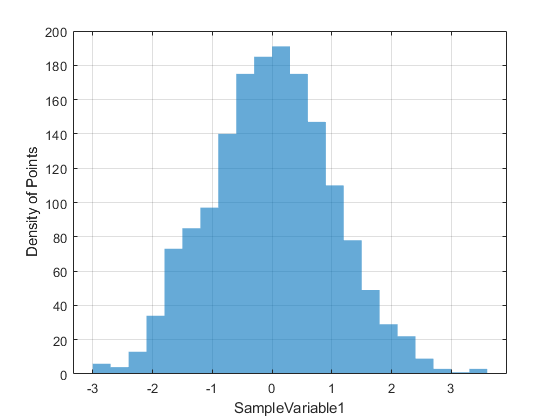If the variable names are specified for the sampler before running the simulations, the sampler will automatically assign names to each variable. To change the x-label, for example, you can try,
sample.plot.histogram.make();
xlabel("Standard Gaussian Random Value");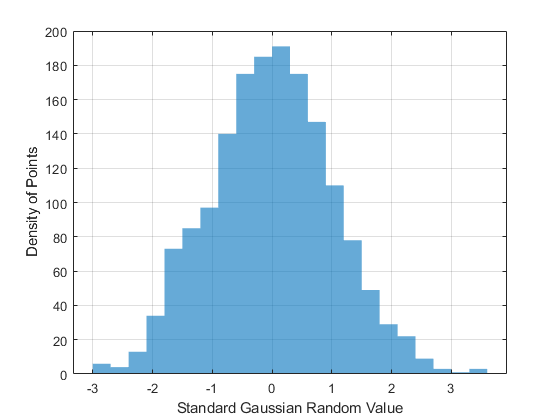To make a trace-plot of the sample, try,
sample.plot.line.make();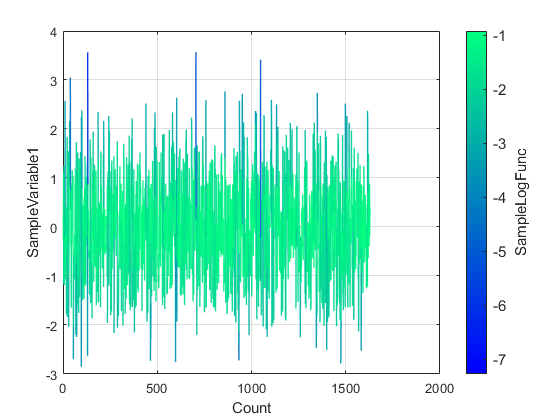Make the trace-plot of the sample on the logarithmic scale, try,
sample.plot.line.make();
set(gca,'xscale','log');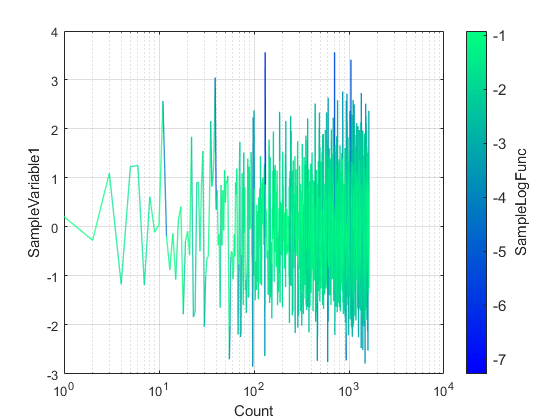There are many other properties of the plot that can be set or modified via the attributes of the sample.plot.line object. To see them all, get help about the object,
sample.plot.line.helpme()
To make a lineScatter plot of the sampled points, try,
sample.plot.lineScatter.scatter.size = 15 % make the dots slightly larger than the default
sample =
TabularFileContents with properties: delimiter: "," file: "D:\temp\libparamonte_MATLAB\out\gaussian_process_1_sample.txt" df: [1629×2 table] plot: [1×1 struct] stats: [1×1 struct] ncol: 2 count: 1629 ndim: 1
sample.plot.lineScatter.make();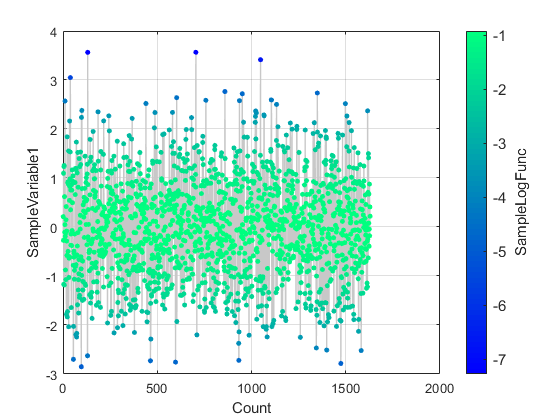Setting or modifying the properties of the scatter plot is highly similar to the procedures used for the line plot. To turn the varying-color off, try,
sample.plot.scatter.reset("hard"); % reset the scatter plot settings to the default.
ParaDRAM - NOTE: creating the scatter plot object from scratch...
sample.plot.scatter.colormap.enabled = false;
sample.plot.scatter.make();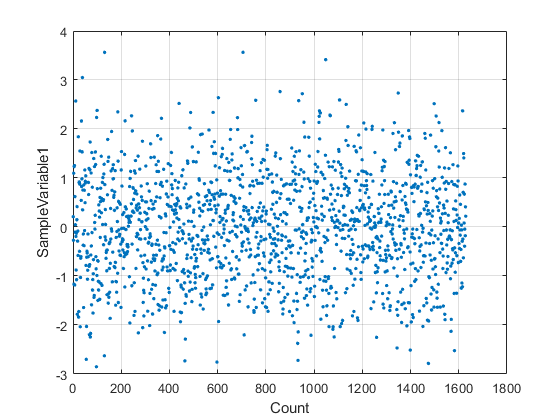Let's make some (2D or 3D) kernel density estimates of the sampled points. To increase the quality of the kernel density estimates, we will use the weighted Markov chain data (in compact format),
chain = chain{1};
chain.plot.contour3.gridSize = 128; % the default is 512
chain.plot.contour3.make();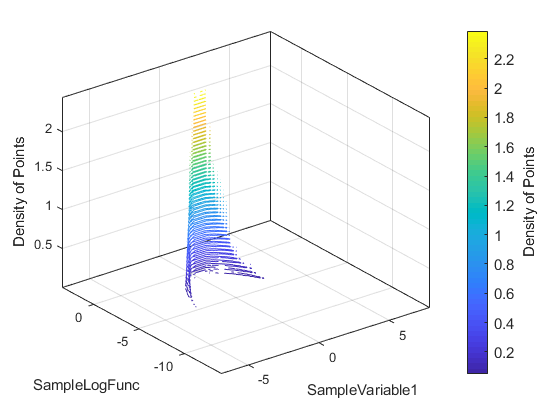Since there is no more than one sampled variable here, the kernel density plot displays SampleLogFunc vs. SampleVariable1.
To visualize the autocorrelation of the raw MCMC chain, try,
markov = markov{1};
markov.stats.autocorr.plot.line.make();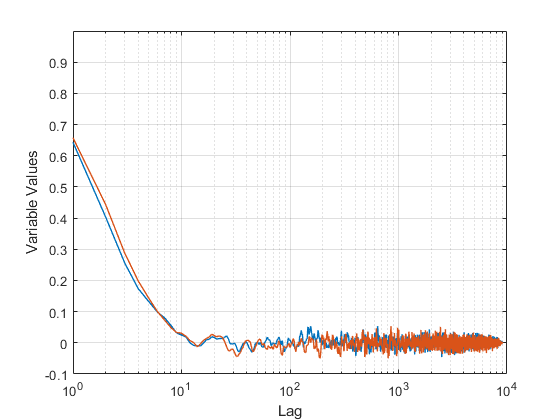Now, for comparison, let's plot the autocorrelation of the final sample, which heavily and agressively refined by the ParaDRAM sampler,
sample.stats.autocorr.plot.line.make();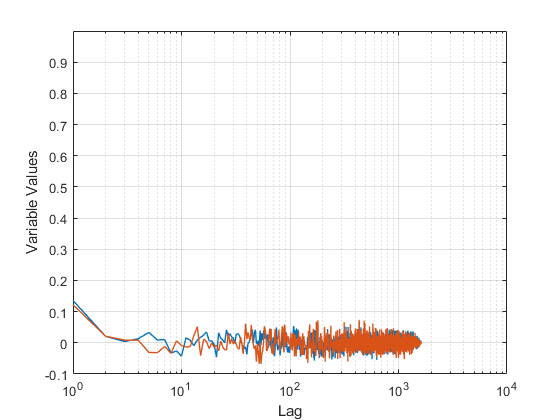The above AutoCorrelation plot for the refined sample is reassuring since the sampled points do not appear to be correlated with each other at all. This is because the ParaDRAM routine, by default, applies as many rounds of refinements and decorrelating of the Markov chain as necessary to remove any residual correlations from the final output random sample.
By contrast, unlike the refined sample, the Markov chain is significantly correlated with itself along each dimension. The large amount of autocorrelation seen for SampleLogFunc is because of the fact that we started the MCMC sampling from a very bad low-probability location, which is also visible the grid plots in the above.

## Final Remarks

=================
There are many more functionalities and features of the ParaMonte library that were neither explored nor mentioned in this example Jupyter notebook. You can explore them by checking the existing components of each attribute of the ParaDRAM sampler class and by visiting the ParaMonte library's documentation website.

{% comment %}
{% endcomment %}